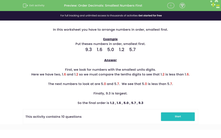# Order Decimals: Smallest Numbers First

In this worksheet, students arrange simple decimal numbers in ascending order.Key stage:  KS 2

Curriculum topic:   Number: Number and Place Value

Curriculum subtopic:   Solve Number Problems to 1000

Difficulty level:#### Worksheet Overview

In this activity we will learn to arrange decimal numbers in order, smallest first.

We are going to be comparing and ordering decimal numbers with one decimal place.

Look at this set of numbers.

7.1    2.3    6.0    2.2    6.4

Firstly, we compare the numbers by how many ones they have.

2.3 and 2.2 both have two ones. These will be the smallest numbers.

Now, we can compare these numbers by how many tenths they have.

2.2 has two tenths and 2.3 has three-tenths.

So, 2.2 is the smallest number.

Next, we can compare 6.0 and 6.4 in the same way. They both have six ones.

6.0 has zero tenths and 6.4 has four-tenths.

So, 6.0 is smaller than 6.4.

Lastly, that leaves us with 7.1, which has seven ones. This is the largest number.

So, the numbers in order are:

2.2    2.3    6.0    6.4    7.1

Now try an example question:

Example

Put these numbers in order, smallest first.

9.3    1.6    5.0    1.2    5.7

First, we look for numbers with the smallest ones digits.

Here we have two, 1.6 and 1.2 so we must compare the tenths digits to see that 1.2 is less than 1.6.

The next numbers to look at are 5.0 and 5.7.  We see that 5.0 is worth less than 5.7.

Finally, 9.3 is largest.

So the final order is 1.2 , 1.6 , 5.0 , 5.7 , 9.3

Now you're ready to try some more questions. Good luck!

### What is EdPlace?

We're your National Curriculum aligned online education content provider helping each child succeed in English, maths and science from year 1 to GCSE. With an EdPlace account you’ll be able to track and measure progress, helping each child achieve their best. We build confidence and attainment by personalising each child’s learning at a level that suits them.

Get started